## 1 Introduction

Uniform interpolants were originally studied in the context of non-classical logics, starting from the pioneering work by Pitts . We briefly recall what uniform interpolants are. We fix a logic or a theory T and a suitable fragment L (propositional, first-order quantifier-free, etc.) of its language. Given an L-formula $$\phi (\underline{x}, \underline{y})$$ (where $$\underline{x},\underline{y}$$ are the variables occurring free in $$\phi$$), a uniform interpolant of $$\phi$$ (w.r.t. $$\underline{y}$$) is an L-formula $$\phi '(\underline{x})$$ where only variables $$\underline{x}$$ occur free, and that satisfies the following two properties: (i) $$\phi (\underline{x}, \underline{y})\vdash _T \phi '(\underline{x})$$; (ii) for any further L-formula $$\psi (\underline{x}, \underline{z})$$ such that $$\phi (\underline{x}, \underline{y}) \vdash _T \psi (\underline{x}, \underline{z})$$, we have $$\phi '(\underline{x}) \vdash _T \psi (\underline{x}, \underline{z})$$. Whenever uniform interpolants exist, one can compute an interpolant for an entailment like $$\phi (\underline{x}, \underline{y}) \vdash _T \psi (\underline{x}, \underline{z})$$ in such a way that this interpolant is independent of $$\psi$$.

The existence of uniform interpolants is an exceptional phenomenon, which is however not so infrequent; it has been extensively studied in non-classical logics starting from the nineties, as witnessed by a large literature, including for instance [7, 22, 33,34,35, 43, 58, 62, 63]). The main results from the above papers are that uniform interpolants exist for intuitionistic logic and for some modal systems (like the Gödel-Lob system and the S4.Grz system); they do not exist for instance in S4 and K4, whereas for the basic modal system K they exist for the local consequence relation but not for the global consequence relation.

In the last decade, also the automated reasoning community developed an increasing interest in uniform interpolants, with particular focus on quantifier-free fragments of first-order theories. This is witnessed by various talks and drafts by Kapur presented in many conferences and workshops (FloC 2010, ISCAS 2013-14, SCS 2017 ), as well as by the paper presented in ESOP 2008 authored by Gulwani and Musuvathi . In this last paper uniform interpolants were renamed as covers, a terminology we shall adopt in this paper too. In these contributions, examples of cover computations were supplied and also some algorithms were sketched. The first formal proof about the existence of covers in $$\mathcal {EUF}$$ was however published only in  by the present authors; such a proof was equipped with powerful semantic tools (see the Cover-by-Extensions Lemma 3.1 below) obtained thanks to interesting connections with model completeness , and came with an algorithm for computing covers that is based on a constrained variant of the Superposition Calculus . Both the model-theoretic tools and the algorithm are detailed in the present paper. Two simple additional algorithms, which exploit DAG representations of terms, are studied in [26, 27].

The usefulness of covers in model checking was already stressed in  and further motivated by our recent line of research on the verification of data-aware processes (also called ‘database driven applications’ in this paper) [12, 13, 15, 17]. Notably, this is also operationally mirrored in the MCMT model checker  starting from version 2.8 (database driven module). The need for incorporating this algorithm within MCMT is due to the following reason. Declarative approaches to infinite state model checking  need to manipulate logical formulae in order to represent sets of reachable states. To prevent divergence, various abstraction strategies have been adopted, ranging from interpolation-based  to sophisticated search via counterexample elimination . Precise computations of the set of reachable states require some form of quantifier elimination and hence are subject to two problems, namely that quantifier elimination might not be available at all and that, when available, it is computationally very expensive. To cope with the first problem, Gulwani and Musuvathi  introduced the notion of cover and showed that covers can be used as an alternative to quantifier elimination and yield a precise computation of reachable states. Concerning the second problem, again in  it was observed (as a side remark) that computing the cover of a conjunction of literals becomes tractable when only free unary function symbols occur in the signature. We show here (see Sect. 6 below) that the same observation applies when also free relational symbols occur.

In [11, 13] we propose a new formalism for representing read-only database schemas towards the verification of integrated models of processes and data  (called data-aware processes henceforth), in particular so-called artifact systems [8, 20, 44, 61]; this formalism (briefly recalled in Sect. 4.1 below) precisely uses signatures comprising unary function symbols and free n-ary relations. In [11, 13, 17] we apply model completeness techniques for verifying transition systems based on read-only databases, in a framework where such systems employ both individual and higher order variables.

In this paper we show (see Sect. 3 below) that covers (alias uniform interpolants) are strictly related to model completions, thus creating a bridge that links different research areas. In particular, we prove that computing covers for a theory is equivalent to eliminating quantifiers in its model completion. This connection reproduces, in a first-order setting, an analogous well-known connection for propositional logics: the connection between propositional uniform interpolants and model completions of equational theories axiomatizing the varieties corresponding to propositional logics, which was first stated in  and further developed in [33, 43, 62]. Interestingly, model completeness has other well-known applications in computer science. It has been applied: (i) to reveal interesting connections between temporal logic and monadic second-order logic [29, 30]; (ii) in automated reasoning to design complete algorithms for constraint satisfiability in combined theories over non-disjoint signatures [1, 23, 31, 49,50,51]; (iii) again in automated reasoning in relationship with interpolation and symbol elimination [59, 60]; (iv) in modal logic and in software verification theories [24, 25], to obtain combined interpolation results.

This paper is organized as follows. After some preliminaries in Sect. 2, we first state the formal connection between uniform quantifier-free interpolation and model completions in Sect. 3. Then, in Sect. 4 we report our applications (mostly taken from ) concerning verification of data-aware processes. We begin the second part of the paper by proving (Sect. 5 below) that covers for $$\mathcal {EUF}$$ can be computed through a constrained version of the Superposition Calculus  equipped with appropriate settings and reduction strategies; the related completeness proof requires a careful analysis of the constrained literals generated during the saturation process. Complexity bounds for the fragment used in data-aware processes verification are investigated in Sect. 6; an extension of our constrained Superposition Calculus that handles a schema of additional constraints (useful for our applications) is provided in Sect. 7; in Sect. 8 we give some details about our first implementation in our tool mcmt. This paper is the extended version of : apart from containing more basic preliminary material, a thorough account of model-checking applications, full proofs and detailed examples, in Sects. 6 and 7 this paper covers additional new results on complexity analysis and extensions.

## 2 Preliminaries

We adopt the usual first-order syntactic notions of signature, term, atom, (ground) formula, and so on; our signatures are multi-sorted and include equality for every sort. This implies that variables are sorted as well. For simplicity, most basic definitions in this section will be supplied for single-sorted languages only. However, the adaptation to multi-sorted languages is straightforward: for example, a multi-sorted signature $$\varSigma$$ must contain not only constant, function and relation symbols, but also sorts. We compactly represent a tuple $$\langle x_1,\ldots ,x_n\rangle$$ of variables as $$\underline{x}$$. The notation $$t(\underline{x}), \phi (\underline{x})$$ means that the term t, the formula $$\phi$$ has free variables included in the tuple $$\underline{x}$$. Our tuples are assumed to be formed by distinct variables, thus we underline that, writing e.g. $$\phi (\underline{x}, \underline{y})$$, we mean that the tuples $$\underline{x}, \underline{y}$$ are made of distinct variables that are also disjoint from each other.

We assume that a function arity can be deduced from the context. Whenever we build terms and formulae, we always assume that they are well-typed, in the sense that the sorts of variables, constants, and function sources/targets match. A formula is said to be universal (resp., existential) if it has the form $$\forall \underline{x}(\phi (\underline{x}))$$ (resp., $$\exists \underline{x}(\phi (\underline{x}))$$), where $$\phi$$ is a quantifier-free formula. Formulae with no free variables are called sentences.

From the semantic side, we use the standard notion of a $$\varSigma$$-structure $$\mathcal M$$ and of truth of a formula in a $$\varSigma$$-structure under a free variables assignment. The support of a structure $$\mathcal M$$ is the disjoint union of the interpretations of the $$\varSigma$$-sorts in $$\mathcal M$$ and is indicated with $$\vert \mathcal M\vert$$.

A $$\varSigma$$-theory T is a set of $$\varSigma$$-sentences; a model of T is a $$\varSigma$$-structure $$\mathcal M$$ where all sentences in T are true. We use the standard notation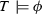to say that $$\phi$$ is true in all the models of T for every assignment to the variables occurring free in $$\phi$$. We say that $$\phi$$ is T-satisfiable iff there is a model $$\mathcal M$$ of T and an assignment to the variables occurring free in $$\phi$$ making $$\phi$$ true in $$\mathcal M$$.

A $$\varSigma$$-formula $$\phi$$ is a $$\varSigma$$-constraint (or just a constraint) iff it is a conjunction of literals, i.e. of atomic formulae and their negations.

The constraint satisfiability problem for T is the following: we are given a constraint (equivalently, a quantifier-free formula) $$\phi (\underline{x})$$

and we are asked whether there exist a model $$\mathcal M$$ of T and an assignment $$\mathcal I$$ to the free variables $$\underline{x}$$ such that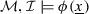.

A theory T has quantifier elimination iff for every formula $$\phi (\underline{x})$$ in the signature of T there is a quantifier-free formula $$\phi '(\underline{x})$$ such that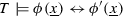. It is well-known (and easily seen) that quantifier elimination holds in case we can eliminate quantifiers from primitive formulae, i.e. from formulae of the kind $$\exists \underline{y}\,\phi (\underline{x}, \underline{y})$$, where $$\phi$$ is a constraint. Since we are interested in effective computability, we assume that when we talk about quantifier elimination, an effective procedure for eliminating quantifiers is given.

We recall also some basic definitions and notions from model theory.

Let $$\varSigma$$ be a first-order signature. The signature obtained from $$\varSigma$$ by adding to it a set $$\underline{a}$$ of new constants (i.e., 0-ary function symbols) is denoted by $$\varSigma ^{\underline{a}}$$. Analogously, given a $$\varSigma$$-structure $$\mathcal M$$, the signature $$\varSigma$$ can be expanded to a new signature $$\varSigma ^{|\mathcal M|}:=\varSigma \cup \{\bar{a}\ |\ a\in |\mathcal M| \}$$ by adding a set of new constants $$\bar{a}$$ (the name for a), one for each element a in $$\mathcal M$$, with the convention that two distinct elements are denoted by different “name” constants. $$\mathcal M$$ can be expanded to a $$\varSigma ^{|\mathcal M|}$$-structure just interpreting the additional constants over the corresponding elements. From now on, we confuse $$\mathcal M$$ and this expanded structure and we do not distinguish from an element of $$|\mathcal M|$$ and its name. Thus we employ notations like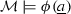to mean that the sentence $$\phi (\underline{a})$$ (obtained by replacing the free variables $$\underline{x}$$ of $$\phi (\underline{x})$$ by the names of some tuple $$\underline{a}$$ from $$|\mathcal M|$$) is true in $$\mathcal M$$, once $$\mathcal M$$ is canonically expanded to a $$\varSigma ^{|\mathcal M|}$$-structure as explained above. Notice that this is the same as saying that $$\phi (\underline{x})$$ is true in $$\mathcal M$$ under the assignment mapping the $$\underline{x}$$ to the $$\underline{a}$$.

A $$\varSigma$$-embedding  (or, simply, an embedding) between two $$\varSigma$$-structures $$\mathcal M$$ and $$\mathcal N$$ is a map $$\mu : \vert \mathcal M\vert \longrightarrow \vert \mathcal N\vert$$ among the support sets $$\vert \mathcal M\vert$$ of $$\mathcal M$$ and $$\vert \mathcal N\vert$$ of $$\mathcal N$$ satisfying the condition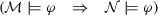for all $$\varSigma ^{\vert \mathcal M\vert }$$-literals $$\varphi$$ ($$\mathcal M$$ is regarded as a $$\varSigma ^{\vert \mathcal M\vert }$$-structure, by interpreting each additional constant $$a\in \vert \mathcal M\vert$$ into itself and $$\mathcal N$$ is regarded as a $$\varSigma ^{\vert \mathcal M\vert }$$-structure by interpreting each additional constant $$a\in \vert \mathcal M\vert$$ into $$\mu (a)$$). If $$\mu : \mathcal M\longrightarrow \mathcal N$$ is an embedding that is just the identity inclusion $$\vert \mathcal M\vert \subseteq \vert \mathcal N\vert$$, we say that $$\mathcal M$$ is a substructure of $$\mathcal N$$ or that $$\mathcal N$$ is an extension of $$\mathcal M$$. We recall that a substructure preserves and reflects validity of ground formulae, in the following sense: given a $$\varSigma$$-substructure $$\mathcal M_1$$ of a $$\varSigma$$-structure $$\mathcal M_2$$, a ground $$\varSigma ^{\vert \mathcal M_1\vert }$$-sentence $$\theta$$ is true in $$\mathcal M_1$$ iff $$\theta$$ is true in $$\mathcal M_2$$.

Let $$\mathcal M$$ be a $$\varSigma$$-structure. The diagram of $$\mathcal M$$, written $$\varDelta _{\varSigma }(\mathcal M)$$ (or just $$\varDelta (\mathcal M)$$), is the set of ground $$\varSigma ^{|\mathcal M|}$$-literals that are true in $$\mathcal M$$. An easy but important result, called Robinson Diagram Lemma , says that, given any $$\varSigma$$-structure $$\mathcal N$$, the embeddings $$\mu : \mathcal M\longrightarrow \mathcal N$$ are in bijective correspondence with expansions of $$\mathcal N$$ to $$\varSigma ^{\vert \mathcal M\vert }$$-structures which are models of $$\varDelta _{\varSigma }(\mathcal M)$$. The expansions and the embeddings are related in the obvious way: $${\bar{a}}$$ is interpreted as $$\mu (a)$$. The typical use of the Robinson Diagram Lemma is the following: suppose we want to show that some structure $$\mathcal M$$ can be embedded into a structure $$\mathcal N$$ in such a way that some set of sentences $$\varDelta$$ are true. Then, by the Lemma, this turns out to be equivalent to the fact that the set of sentences $$\varDelta (\mathcal M)\cup \varDelta$$ is consistent: thus, the Diagram Lemma can be used to transform an embeddability problem into a consistency problem (the latter is a problem of a logical nature, to be solved for instance by appealing to the compactness theorem for first-order logic).

Amalgamation is a classical algebraic concept. We give the formal definition:

### Definition 2.1

(Amalgamation) A theory T has the amalgamation property if for every couple of embeddings $$\mu _1:\mathcal M_0\longrightarrow \mathcal M_1$$, $$\mu _2:\mathcal M_0\longrightarrow \mathcal M_2$$ among models of T, there exists a model $$\mathcal M$$ of T endowed with embeddings $$\nu _1:\mathcal M_1 \longrightarrow \mathcal M$$ and $$\nu _2:\mathcal M_2 \longrightarrow \mathcal M$$ such that $$\nu _1\circ \mu _1=\nu _2\circ \mu _2$$. $$\lhd$$

## 3 Covers, Uniform Interpolation and Model Completions

We report the notion of cover taken from . Fix a theory T and an existential formula $$\exists \underline{e}\, \phi (\underline{e}, \underline{y})$$; call a residue of $$\exists \underline{e}\, \phi (\underline{e}, \underline{y})$$ any quantifier-free formula belonging to the set of quantifier-free formulae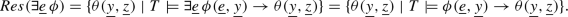A quantifier-free formula $$\psi (\underline{y})$$ is said to be a T-cover (or, simply, a cover) of $$\exists \underline{e}\, \phi (\underline{e},\underline{y})$$ iff $$\psi (\underline{y})\in Res(\exists \underline{e}\, \phi )$$ and $$\psi (\underline{y})$$ implies (modulo T) all the other formulae in $$Res(\exists \underline{e}\, \phi )$$. Notice that the cover is unique, modulo T-equivalence. Alternatively, $$\psi (\underline{y})$$ is also said to be a T-uniform (quantifier-free) interpolant of $$\phi (\underline{e},\underline{y})$$. The following Lemma (to be widely used throughout the paper) supplies a semantic counterpart to the notion of a cover:

### Lemma 3.1

(Cover-by-Extensions) A formula $$\psi (\underline{y})$$ is a T-cover of $$\exists \underline{e}\, \phi (\underline{e}, \underline{y})$$ iff it satisfies the following two conditions: (i)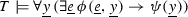; (ii) for every model $$\mathcal M$$ of T, for every tuple of elements $$\underline{a}$$ from the support of $$\mathcal M$$ such that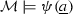it is possible to find another model $$\mathcal N$$ of T such that $$\mathcal M$$ embeds into $$\mathcal N$$ and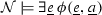. $$\lhd$$

### Proof

Suppose that $$\psi (\underline{y})$$ satisfies conditions (i) and (ii) above. Condition (i) says that $$\psi (\underline{y})\in Res(\exists \underline{e}\, \phi )$$, so $$\psi$$ is a residue. In order to show that $$\psi$$ is also a cover, we have to prove that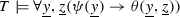, for every $$\theta (\underline{y},\underline{z})$$ that is a residue for $$\exists \underline{e}\, \phi (\underline{e}, \underline{y})$$. Given a model $$\mathcal M$$ of T, take a pair of tuples $$\underline{a}, \underline{b}$$ of elements from $$|\mathcal M|$$ and suppose that. By condition (ii), there is a model $$\mathcal N$$ of T such that $$\mathcal M$$ embeds into $$\mathcal N$$ and. Using the definition of $$Res(\exists \underline{e}\, \phi )$$, we have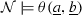, since $$\theta (\underline{y},\underline{z})\in Res(\exists \underline{x}\, \phi )$$. Since $$\mathcal M$$ is a substructure of $$\mathcal N$$ and $$\theta$$ is quantifier-free,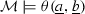as well, as required.

Suppose that $$\psi (\underline{y})$$ is a cover. The definition of residue implies condition (i). To show condition (ii) we have to prove that, given a model $$\mathcal M$$ of T, for every tuple $$\underline{a}$$ of elements from $$|\mathcal M|$$, if, then there exists a model $$\mathcal N$$ of T such that $$\mathcal M$$ embeds into $$\mathcal N$$ and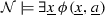. Using Robinson Diagram Lemma, we can reformulate the latter embeddability statement into a consistency statement: so what we need to prove is that $$\varDelta (\mathcal M)\cup \{ \exists \underline{x}\, \phi (\underline{x}, \underline{a}) \}$$ is a T-consistent $$\varSigma ^{|\mathcal M|}$$-set of sentences ($$\varSigma$$ is the signature of T). By reduction to absurdity, suppose that this is not the case: by compactness, there is a finite number of literals $$\ell _1(\underline{a},\underline{b}),...,\ell _m(\underline{a},\underline{b})$$ (for some tuple $$\underline{b}$$ of elements from $$|\mathcal M|$$) such that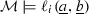(for all $$i=1,\dots ,m$$) and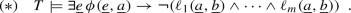Now, the constants $$\underline{a},\underline{b}$$ do not occur in the axioms of T and do not belong to $$\varSigma$$, hence we can replace them by variables $$\underline{y}, \underline{z}$$ in the T-proof witnessing $$(*)$$: indeed, since they do not occur in the axioms of T, they are generic from the point of view of T. As a consequence, we then get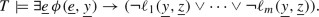By definition of residue, clearly $$(\lnot \ell _1(\underline{y},\underline{z})\vee \dots \vee \lnot \ell _m(\underline{y},\underline{z})) \in Res(\exists \underline{x}\, \phi )$$; then, since $$\psi (\underline{y})$$ is a cover,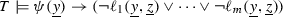. Replacing back the variables $$\underline{y}, \underline{z}$$ by the constants $$\underline{a}, \underline{b}$$ and recalling that, this implies that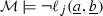for some $$j=1,\dots ,m$$, which is a contradiction. Thus, $$\psi (\underline{y})$$ satisfies conditions (ii) too. $$\square$$

We say that a theory T has uniform quantifier-free interpolation iff every existential formula $$\exists \underline{e}\, \phi (\underline{e},\underline{y})$$ (equivalently, every primitive formula $$\exists \underline{e}\, \phi (\underline{e},\underline{y})$$) has a T-cover. It is clear that if T has uniform quantifier-free interpolation, then it has ordinary quantifier-free interpolation , in the sense that if we have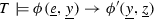(for quantifier-free formulae $$\phi , \phi '$$), then there is a quantifier-free formula $$\theta (\underline{y})$$ such that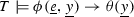and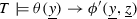. In fact, if T has uniform quantifier-free interpolation, then the interpolant $$\theta$$ is independent on $$\phi '$$: indeed, the same $$\theta (\underline{y})$$ can be used as interpolant for all entailments, varying $$\phi '$$.

We say that a universal theory T has a model completion iff there is a stronger theory $$T^*\supseteq T$$ (still within the same signature $$\varSigma$$ of T) such that (i) every $$\varSigma$$-constraint that is satisfiable in a model of T is satisfiable in a model of $$T^*$$; (ii) $$T^*$$ eliminates quantifiers. Other equivalent definitions are possible : for instance, (i) is equivalent to the fact that T and $$T^*$$ prove the same quantifier-free formulae or again to the fact that every model of T can be embedded into a model of $$T^*$$. We recall that the model completion, if it exists, is unique and that its existence implies the amalgamation property for T . The relationship between uniform interpolation in a propositional logic and the model completion

of the equational theory of the variety algebraizing it was extensively studied in . In the context of first-order theories, we prove an even more direct connection:

### Theorem 3.2

Suppose that T is a universal theory. Then T has a model completion $$T^*$$ iff T has uniform quantifier-free interpolation. If this happens, $$T^*$$ is axiomatized by the infinitely many sentences

\begin{aligned} \forall \underline{y}\,(\psi (\underline{y}) \rightarrow \exists \underline{e}\, \phi (\underline{e}, \underline{y})) \end{aligned}
(1)

where $$\exists \underline{e}\, \phi (\underline{e}, \underline{y})$$ is a primitive formula and $$\psi$$ is a cover of it. $$\lhd$$

### Proof

Suppose first that there is a model completion $$T^*$$ of T and let $$\exists \underline{e}\,\phi (\underline{e},\underline{y})$$ be a primitive formula. Since $$T^*$$ eliminates quantifiers, we have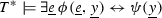for some quantifier-free formula $$\psi (\underline{y})$$. Since T and $$T^*$$ prove the same quantifier-free formulae, from the left-to-right side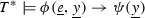we have that $$\psi (\underline{y})\in Res(\exists \underline{e}\,\phi )$$. If $$\theta (\underline{y},\underline{z})\in Res(\exists \underline{e}\,\phi )$$, then we have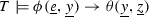; the same entailment holds in $$T^*$$ too, where we have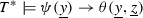. Since $$\psi (\underline{y})\rightarrow \theta (\underline{y},\underline{z})$$ is quantifier-free, we have also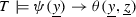, showing that $$\psi$$ is a cover of $$\exists \underline{e}\,\phi (\underline{e},\underline{y})$$. Thus T has uniform interpolation, because we found a cover for every primitive formula.

Suppose vice versa that T has uniform interpolation. Let $$T^*$$ be the theory axiomatized by all the formulae (1) above.

From (i) of Lemma 3.1 and (1) above, we clearly get that $$T^{\star }$$ admits quantifier elimination: in fact, in order to prove that a theory enjoys quantifier elimination, it is sufficient to eliminate quantifiers from primitive formulae (then the quantifier elimination for all formulae can be easily shown by an induction over their complexity). This is exactly what is guaranteed by (i) of Lemma 3.1 and (1).

Let $$\mathcal M$$ be a model of T. By using a chain argument  (see , Lemma 3.5.7 for an almost identical construction), we show that there exists a model $$\mathcal M^{\prime }$$ of $$T^{\star }$$ such that $$\mathcal M$$ embeds into $$\mathcal M^{\prime }$$.

Consider the set of all pairs $$(\underline{a}, \exists \underline{e}\,\phi (\underline{e}, \underline{a}))$$ where $$\underline{a}$$ is a tuple from $$|\mathcal M|$$, $$\exists \underline{e}\,\phi (\underline{e},\underline{y})$$ is a primitive formula and(here $$\psi$$ is a cover of $$\phi$$). By Zermelo’s Theorem, the set of such pairs $$(\underline{a}, \exists \underline{e}\,\phi (\underline{e}, \underline{a}))$$ can be well-ordered: let $$\{(\underline{a}_i,\exists \underline{e}_i\,\phi _i(\underline{e}_i, \underline{a}_i))\}_{i\in I}$$ be such a well-ordered set of pairs, where I is some ordinal.Footnote 1 By transfinite induction on this well-order, we define $$\mathcal M_{0}:=\mathcal M$$ and, for each $$i\in I$$, $$\mathcal M_{i}$$ as an extension of $$\bigcup _{j<i}\mathcal M_j$$ such that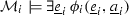, which exists for (ii) of Lemma 3.1 since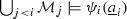(remember that validity of ground formulae is preserved passing through substructures and superstructures, and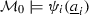).

Now we take the chain union $$\mathcal M^{1}:=\bigcup _{i\in I} \mathcal M_{i}$$: since T is universal, $$\mathcal M^{1}$$ is again a model of T. Thanks to this construction, we added, for every pair $$(\underline{a}_i,\exists \underline{e}_i\,\phi _i(\underline{e}_i, \underline{a}_i))$$ (with $$\underline{a}_i \in \mathcal M$$ and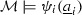), a corresponding tuple $$\underline{b}_i$$ such that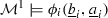; however, this only guarantees that such a tuple $$\underline{b}_i$$ exists for every pair $$(\underline{a}_i,\exists \underline{e}_i\,\phi _i(\underline{e}_i, \underline{a}_i))$$ such that the tuple $$\underline{a}_i$$ is from $$|\mathcal M|$$, whereas nothing is said for the pairs where the tuple $$\underline{a}$$ is in $$|\mathcal M^{1}|\setminus |\mathcal M|$$. Then, we iteratively repeat the chain construction above for these new $$\underline{a}$$. Indeed, it is possible to construct, by an analogous chain argument, a model $$\mathcal M^{2}$$ as done above, starting from $$\mathcal M^{1}$$ instead of $$\mathcal M$$. Clearly, we get $$\mathcal M_{0}:=\mathcal M\subseteq \mathcal M^{1} \subseteq \mathcal M^2$$ by construction.

At this point, we iterate the same argument countably many times, so as to define a new chain of models of T:

\begin{aligned} \mathcal M_{0}:=\mathcal M\subseteq \mathcal M^{1}\subseteq ...\subseteq \mathcal M^n\subseteq ... \end{aligned}

Defining $$\mathcal M^{\prime }:=\bigcup _n \mathcal M^{n}$$, we trivially get that $$\mathcal M^{\prime }$$ is a model of T such that $$\mathcal M\subseteq \mathcal M^{\prime }$$ and satisfies all the sentences of type (1): the last fact is immediate, recalling that truth of ground formulae (in expanded languages with names from support sets) is preserved by substructures and extensions. After $$\omega$$ steps we are done, because every tuple $$\underline{a}\in |\mathcal M^{\prime }|$$ occurs after finitely many steps, and its corresponding $$\underline{b}$$ in the construction are added at the immediately subsequent step. $$\square$$

To sum up, Theorem 3.2 states that, thanks to Formulae (1), the T-uniform interpolant (or cover) $$\psi$$ of the formula $$\exists \underline{e}\, \phi (\underline{e}, \underline{y})$$ is exactly the $$T^*$$-equivalent quantifier-free formula that eliminates the quantified variables $$\underline{e}$$ from $$\exists \underline{e}\, \phi (\underline{e}, \underline{y})$$: this means that computing covers in T is equivalent to eliminating quantifiers in its model completion $$T^*$$.

## 4 Model-Checking Applications

In this section we supply old and new motivations for investigating covers and model completions in view of model-checking applications. We first report the considerations from [11, 13, 17, 37] on symbolic model-checking via model completions (or, equivalently, via covers) in the basic case where system variables are represented as individual variables; for more advanced applications where system variables are both individual and higher order variables, see [11, 13, 17]. Similar ideas (i.e., ‘to use quantifier elimination in the model completion even if T does not allow quantifier elimination’) were used in  for interpolation and symbol elimination.

### Definition 4.1

A (quantifier-free) transition system is a tuple

\begin{aligned} \mathcal S~=~\langle \varSigma , T, \underline{x}, \iota (\underline{x}), \tau (\underline{x}, \underline{x}')\rangle \end{aligned}

where: (i) $$\varSigma$$ is a signature and T is a $$\varSigma$$-theory; (ii) $$\underline{x}=x_1, \dots , x_n$$ are individual variables; (iii) $$\iota (\underline{x})$$ is a quantifier-free formula; (iv) $$\tau (\underline{x}, \underline{x}')$$ is a quantifier-free formula (here the $$\underline{x}'$$ are renamed copies of the $$\underline{x}$$). $$\lhd$$

A safety formula for a transition system $$\mathcal S$$ is a further quantifier-free formula $$\upsilon (\underline{x})$$ describing undesired states of $$\mathcal S$$. We say that $$\mathcal S$$ is safe with respect to $$\upsilon$$ if the system has no finite run leading from $$\iota$$ to $$\upsilon$$, i.e. (formally) if there is no model $$\mathcal M$$ of T and no $$k\ge 0$$ such that the formula

\begin{aligned} \iota (\underline{x}^0) \wedge \tau (\underline{x}^0, \underline{x}^1) \wedge \cdots \wedge \tau (\underline{x}^{k-1}, \underline{x}^k)\wedge \upsilon (\underline{x}^k) \end{aligned}
(2)

is satisfiable in $$\mathcal M$$ (here $$\underline{x}^i$$’s are renamed copies of $$\underline{x}$$). The safety problem for $$\mathcal S$$ is the following: given $$\upsilon$$, decide whether $$\mathcal S$$ is safe with respect to $$\upsilon$$.

Suppose now that the theory T mentioned in Definition 4.1 (i) is universal, has decidable constraint satisfability problem and admits a model completion $$T^*$$. Algorithm 1 describes the backward reachability algorithm for handling the safety problem for $$\mathcal S$$ (the dual algorithm working via forward search is described in equivalent terms in ). An integral part of the algorithm is to compute preimages. For that purpose, for any $$\varphi _1(\underline{x},\underline{x}')$$ and $$\varphi _2(\underline{x})$$ (where $$\underline{x}'$$ are renamed copies of $$\underline{x}$$), we define $$Pre (\varphi _1,\varphi _2)$$ to be the formula $$\exists \underline{x}'(\varphi _1(\underline{x}, \underline{x}')\wedge \varphi _2(\underline{x}'))$$.

The preimage of the set of states described by a state formula $$\phi (\underline{x})$$ is the set of states described by $$Pre (\tau ,\phi )$$. The subprocedure $${\mathsf {Q}}{\mathsf {E}}(T^*,\phi )$$ in Line 6 applies the quantifier elimination algorithm of $$T^*$$ to the existential formula $$\phi$$. Without the application of this subprocedure, the existential prefix generated by the computation of preimages would grow in an unlimited way and some decidability results (see, e.g., the locally finite case mentioned below) would be compromized. Algorithm 1 computes iterated preimages of $$\upsilon$$ (storing their disjunction into the variable B) and applies to them quantifier elimination, until a fixpoint is reached or until a set intersecting the initial states (i.e., satisfying $$\iota$$) is found. Inclusion (Line 2) and disjointness (Line 3) tests produce proof obligations that can be discharged because T has decidable constraint satisfiability problem.

The proof of Proposition 4.2 (which is a slight variant of a similar result for Simple Artifact Systems (SASs) in ) consists just in the observation that the formulae (2) are quantifier-free and that a quantifier-free formula is satisfiable in a model of T iff so is it in a model of $$T^*$$: thus, if an unsafe trace exists at all, it arises in a model of $$T^*$$, so that the subprocedure $${\mathsf {Q}}{\mathsf {E}}(T^*,\phi )$$ in Line 6 does not introduce overapproximations and consequently no spurious trace can be produced during the search performed by our algorithm.

### Proposition 4.2

Suppose that the universal $$\varSigma$$-theory T has decidable constraint satisfiability problem and admits a model completion $$T^*$$. For every transition system $$\mathcal S=\langle \varSigma ,T,\underline{x},\iota ,\tau \rangle$$, the backward search algorithm is effective and partially correct for solving safety problems for $$\mathcal S$$.Footnote 2$$\lhd$$

Despite its simplicity, Proposition 4.2 is a crucial fact. Notice that it implies decidability of the safety problems in some interesting cases: this happens, for instance, when in T there are only finitely many quantifier-free formulae in which $$\underline{x}$$ occur, as in case T has a purely relational signature or, more generally, T is locally finiteFootnote 3. Since a theory is universal iff it is closed under substructures  and since a universal locally finite theory has a model completion iff it has the amalgamation property [45, 64], it follows that Proposition 4.2 can be used to cover the decidability result stated in Theorem 5 of  (once restricted to transition systems over a first-order definable class of $$\varSigma$$-structures).

### 4.1 Database Schemas

In this subsection, we provide a new application for the above explained model-checking techniques [13, 17]. The application relates to the verification of integrated models of business processes and data , referred to as artifact systems , where the behavior of the process is influenced by data stored in a relational database (DB) with constraints. The data contained therein are read-only: they can be queried by the process and stored in a working memory, which in the context of this paper is constituted by a set of system variables. In this context, safety amounts to checking whether the system never reaches an undesired property, irrespectively of what is contained in the read-only DB.

We define next the two key notions of (read-only) DB schema and instance, by relying on an algebraic, functional characterization.

### Definition 4.3

A DB schema is a pair $$\langle \varSigma ,T\rangle$$, where: (i) $$\varSigma$$ is a DB signature, that is, a finite multi-sorted signature whose function symbols are all unary; (ii) T is a DB theory, that is, a set of universal $$\varSigma$$-sentences. $$\lhd$$

Given a DB signature $$\varSigma$$, we denote by $$\varSigma _{ srt }$$ the set of sorts, by $$\varSigma _{ fun }$$ the set of functions in $$\varSigma$$ and by $$\varSigma _{ rel }$$ the set of relations in $$\varSigma$$. We associate to a DB signature $$\varSigma$$ a characteristic (directed) graph $$G(\varSigma )$$ capturing the dependencies induced by functions over sorts. Specifically, $$G(\varSigma )$$ is an edge-labeled graph whose set of nodes is $$\varSigma _{ srt }$$, and with a labeled edge $$S \xrightarrow {f} S'$$ for each $$f:S\longrightarrow S'$$ in $$\varSigma _{ fun }$$. We say that $$\varSigma$$ is acyclic if $$G(\varSigma )$$ is so. The leaves of $$\varSigma$$ are the nodes of $$G(\varSigma )$$ without outgoing edges. These terminal sorts are divided in two subsets, respectively representing unary relations and value sorts. Non-value sorts (i.e., unary relations and non-leaf sorts) are called id sorts, and are conceptually used to represent (identifiers of) different kinds of objects. Value sorts, instead, represent datatypes such as strings, numbers, clock values, etc. We denote the set of id sorts in $$\varSigma$$ by $$\varSigma _{ ids }$$, and that of value sorts by $$\varSigma _{ val }$$, hence $$\varSigma _{ srt } = \varSigma _{ ids }\uplus \varSigma _{ val }$$.

Before giving the formal definition of DB instance, we show an interesting example of DB signature inspired by concrete business processes.

### Example 4.1

() The human resource (HR) branch of a company stores the following information inside a relational database: (i) users registered to the company website, who are potentially interested in job positions offered by the company; (ii) the different, available job categories; (iii) employees belonging to HR, together with the job categories they are competent in (in turn indicating which job applicants they could interview).

To formalize these different aspects, we make use of a DB signature $$\varSigma _{ hr }$$ consisting of: (i) four id sorts, used to respectively identify users, employees, job categories, and the competence relationship connecting employees to job categories; (ii) one value sort containing strings used to name users and employees, and describe job categories. In addition, $$\varSigma _{ hr }$$ contains five function symbols mapping: (i) user identifiers to their corresponding names; (ii) employee identifiers to their corresponding names; (iii) job category identifiers to their corresponding descriptions; (iv) competence identifiers to their corresponding employees and job categories.

The characteristic graph of $$\varSigma _{ hr }$$ is shown in Fig. 1 (left part). $$\lhd$$

We now focus on extensional data conforming to a given DB schema.

### Definition 4.4

A DB instance of DB schema $$\langle \varSigma ,T\rangle$$ is a $$\varSigma$$-structure $$\mathcal M$$ such that $$\mathcal M$$ is a model of T.Footnote 4$$\lhd$$

We respectively denote by $$S^\mathcal M$$, $$f^\mathcal M$$, and $$c^\mathcal M$$ the interpretation in $$\mathcal M$$ of the sort S (this is a set), of the function symbol f (this is a set-theoretic function), and of the constant c (this is an element of the interpretation of the corresponding sort). Obviously, $$f^\mathcal M$$ and $$c^\mathcal M$$ must match the sorts declared in $$\varSigma$$. For instance, if the source and the target of f are, respectively, S and U, then the function $$f^\mathcal M$$ has domain $$S^\mathcal M$$ and range $$U^\mathcal M$$.

One might be surprised by the fact that signatures in our DB schemas contain unary function symbols, beside relational symbols. As shown in [11, 13, 17], the algebraic, functional characterization of DB schema and instance can be actually reinterpreted in the classical, relational model so as to reconstruct the requirements posed in . In this last work, the schema of the read-only database must satisfy the following conditions: (i) each relation schema has a single-attribute primary key; (ii) attributes are typed; (iii) attributes may be foreign keys referencing other relation schemas; (iv) the primary keys of different relation schemas are pairwise disjoint.

We now discuss why these requirements are matched by DB schemas.

Definition 4.3 naturally corresponds to the definition of relational database schema with single-attribute primary and foreign keys. To see this, we adopt the named perspective, where each relation schema is defined by a signature containing a relation name and a set of typed attribute names. Let $$\langle \varSigma ,T\rangle$$ be a DB schema. Each sort S from $$\varSigma$$ corresponds to a dedicated relation $$R_S$$ with the following attributes: (i) one identifier attribute $$id_S$$ with type S; (ii) one dedicated attribute $$a_f$$ with type $$S'$$ for every function symbol f from $$\varSigma$$ of the form $$f: S \longrightarrow S'$$.

The fact that $$R_S$$ is constructed starting from functions in $$\varSigma$$ naturally induces corresponding functional dependencies within $$R_S$$, and inclusion dependencies from $$R_S$$ to other relation schemas. In particular, for each non-id attribute $$a_f$$ of $$R_S$$, we get a functional dependency from $$id_S$$ to $$a_f$$. Altogether, such dependencies witness that $$id _S$$ is the primary key of $$R_S$$. In addition, for each non-id attribute $$a_f$$ of $$R_S$$ whose corresponding function symbol f has id sort $$S'$$ as image, we get an inclusion dependency from $$a_f$$ to the id attribute $$id_{S'}$$ of $$R_{S'}$$. This captures that $$a_f$$ is a foreign key referencing $$R_{S'}$$. This view is shown in the following example.

### Example 4.2

The diagram on the right in Fig. 1 graphically depicts the relational view corresponding to the DB signature of Example 4.1. $$\lhd$$

Given a DB instance $$\mathcal {M}$$ of $$\langle \varSigma ,T\rangle$$, its corresponding relational instance $${\mathcal {R}}[{\mathcal M}]$$ is the minimal set satisfying the following property: for every id sort S from $$\varSigma$$, let $$f_1,\ldots ,f_n$$ be all functions in $$\varSigma$$ with domain $$S^{\mathcal M}$$; then, for every identifier $$\texttt {o} \in S^\mathcal {M}$$, $${\mathcal {R}}[{\mathcal M}]$$ contains a labeled fact of the form $$R_S(id_S\,{:}\,\texttt {o}^\mathcal {M},a_{f_1}\,{:}\,f_1(\texttt {o})^\mathcal {M},\ldots ,a_{f_n}\,{:}\,f_n(\texttt {o})^\mathcal {M})$$, where $$attr\,{:}\,\texttt {c}^{\mathcal {M}}$$ means that the element $$\texttt {c}^{\mathcal {M}}$$ corresponds to the attribute attr of the relation $$R_S$$. In addition, $${\mathcal {R}}[{\mathcal M}]$$ contains the tuples from $$r^\mathcal M$$, for every relational symbol r from $$\varSigma$$ (these relational symbols represent plain relations, i.e. those not possessing a key).

We close our discussion by focusing on DB theories. Notice that $$\mathcal {EUF}$$ suffices to handle the sophisticated setting of artifact systems from [13, 17] (e.g., key dependencies). The role of a non-empty DB theory is to encode background axioms to express additional constraints. We illustrate a typical background axiom, required to handle the possible presence of undefined identifiers/values in the different sorts. This, in turn, is essential to capture artifact systems whose working memory is initially undefined, in the style of [21, 44]. To accommodate this, we add to every sort S of $$\varSigma$$ a constant $$\texttt {undef}_S$$ (written by abuse of notation just $$\texttt {undef}$$ from now on), used to specify an undefined value. Then, for each function symbol f of $$\varSigma$$, we can impose additional constraints involving $$\texttt {undef}$$, for example by adding the following axioms to the DB theory:

\begin{aligned} \forall x~(x = \texttt {undef}\leftrightarrow f(x) = \texttt {undef}) \end{aligned}
(3)

This axiom states that the application of f to the undefined value produces an undefined value, and it is the only situation for which f is undefined.

A slightly different approach may handle many undefined values for each sort; the reader is referred to [11, 13, 17] for examples of concrete database instances formalized in our framework. We just point out that in most cases the kind of axioms that we need for our DB theories T are just one-variable universal axioms (like Axioms 3), so that they fit the hypotheses of Proposition 4.5 below.

We are interested in applying the algorithm of Proposition 4.2 to a (non-deterministic) version of Simple Artifact Systems (SASs) , i.e. transition systems $$\mathcal S~= ~\langle \varSigma , T, \underline{x}, \iota (\underline{x}), \tau (\underline{x}, \underline{x}')\rangle$$, where $$\langle \varSigma ,T\rangle$$ is a DB schema in the sense of Definition 4.3. To this aim, it is sufficient to identify a suitable class of DB theories having a model completion and whose constraint satisfiability problem is decidable. A first result in this sense is given below. Given the characteristic graph $$G(\varSigma )$$ of a DB signature $$\varSigma$$, we recall that $$\varSigma$$ is said to be acyclic if $$G(\varSigma )$$ is so.

### Proposition 4.5

 A DB theory T has decidable constraint satisfiability problem and admits a model completion in case it is axiomatized by finitely many universal one-variable formulae and $$\varSigma$$ is acyclic. $$\lhd$$

We omit the proof of the above proposition, because the proposition does not play a role in the following: the proof can be easily obtained by well-known facts from the literature and is nevertheless reported in full detail in . We only report here the algorithm for quantifier elimination in $$T^*$$ suggested by that proof: given a primitive formula $$\exists \underline{e}\,\phi (\underline{e},\underline{y})$$, the output $$\psi (\underline{y})$$ of the algorithm is simply the conjunction of the set of all quantifier-free $$\chi (\underline{y})$$-formulae such that $$\phi (\underline{e},\underline{y})\rightarrow \chi (\underline{y})$$ is a logical consequences of T (they are finitely many - up to T-equivalence - because $$\varSigma$$ is acyclic). We also notice that, since acyclicity of $$\varSigma$$ yields local finiteness, we immediately get as a Corollary the decidability of safety problems for transitions systems based on DB schemas satisfying the hypotheses of the above theorem.

## 5 Covers via Constrained Superposition

Of course, a model completion may not exist at all. Proposition 4.5 shows that it exists in case T is a DB theory axiomatized by universal one-variable formulae and $$\varSigma$$ is acyclic. The second hypothesis is unnecessarily restrictive and the algorithm for quantifier elimination suggested by the proof of Proposition 4.5 is highly impractical: for this reason we are trying a different approach. In this section, we drop the acyclicity hypothesis and examine the case where the theory T is empty and the signature $$\varSigma$$ may contain function symbols of any arity. Covers in this context were shown to exist already in , using an algorithm that, very roughly speaking, determines all the conditional equations that can be derived concerning the nodes of the congruence closure graph. An algorithm for the generation of interpolants, still relying on congruence closure , is sketched in .

We follow a different plan and we want to produce covers (and show that they exist) using saturation-based theorem proving. The natural idea to proceed in this sense is to take the matrix $$\phi (\underline{e},\underline{y})$$ of the primitive formula $$\exists \underline{e}\, \phi (\underline{e}, \underline{y})$$ we want to compute the cover of: this is a conjunction of literals, so we consider each variable as a free constant, we saturate the corresponding set of ground literals and finally we output the literals involving only the $$\underline{y}$$. For saturation, one can use any version of the superposition calculus . However, this procedure for our problem is not sufficient. As a trivial counterexample consider the primitive formula $$\exists e \,(R(e, y_1)\wedge \lnot R(e, y_2))$$: the set of literals $$\{ R(e, y_1), \lnot R(e, y_2)\}$$ is saturated (recall that we view $$e,y_1,y_2$$ as constants), however the formula has a non-trivial cover $$y_1\ne y_2$$ which is not produced by saturation. If we move to signatures with function symbols, the situation is even worse: the set of literals $$\{ f(e,y_1)=y'_1, f(e,y_2)= y'_2\}$$ is saturated but the formula $$\exists e\, (f(e,y_1)=y'_1\wedge f(e,y_2)= y'_2)$$ has the conditional equality $$y_1=y_2\rightarrow y'_1=y'_2$$ as cover. Disjunctions of disequations might also arise: the cover of $$\exists e\, h(e,y_1,y_2)\ne h(e, y'_1,y'_2)$$ (as well as the cover of $$\exists e\, f(f(e,y_1),y_2)\ne f(f(e,y_1'),y_2')$$, see Example 5.5 below) is $$y_1\ne y'_1\vee y_2\ne y'_2$$Footnote 5

Notice that our problem is different from the problem of producing ordinary quantifier-free interpolants via saturation-based theorem proving : for ordinary Craig interpolants, we have as input two quantifier-free formulae $$\phi (\underline{e}, \underline{y}), \phi '(\underline{y}, \underline{z})$$ such that $$\phi (\underline{e},\underline{y})\rightarrow \phi '(\underline{y}, \underline{z})$$ is valid; here we have a single formula $$\phi (\underline{e}, \underline{y})$$ as input and we are asked to find an interpolant which is good for all possible $$\phi '(\underline{y}, \underline{z})$$ such that $$\phi (\underline{e},\underline{y})\rightarrow \phi '(\underline{y}, \underline{z})$$ is valid. Ordinary interpolants can be extracted from a refutation of $$\phi (\underline{e},\underline{y})\wedge \lnot \phi '(\underline{y}, \underline{z})$$, whereas here we are not given any refutation at all (and we are not even supposed to find one).

What we are going to show is that, nevertheless, saturation via superposition can be used to produce covers, if suitably adjusted. In this section we consider signatures with n-ary function symbols (for all $$n\ge 1$$). For simplicity, we omit n-ary relation symbols (they can be easily handled by rewriting $$R(t_1, \dots , t_n)$$ as $$R(t_1, \dots , t_n)=true$$, as customary in the paramodulation literature ).

We are going to compute the cover of a primitive formula $$\exists \underline{e}\,\phi (\underline{e}, \underline{y})$$ to be fixed for the remainder of this section. We call variables $$\underline{e}$$ existential and variables $$\underline{y}$$ parameters. By applying abstraction steps, we can assume that $$\phi$$ is primitive flat, i.e. that it is a conjunction of $$\underline{e}$$-flat literals, defined below. [By an abstraction step we mean replacing $$\exists \underline{e}\,\phi$$ with $$\exists \underline{e}\, \exists e' \,(e'= u\wedge \phi ')$$, where $$e'$$ is a fresh variable and $$\phi '$$ is obtained from $$\phi$$ by replacing some occurrences of a term $$u(\underline{e}, \underline{y})$$ by $$e'$$].

A term or a formula are said to be $$\underline{e}$$-free iff the existential variables do not occur in it. An $$\underline{e}$$-flat term is an $$\underline{e}$$-free term $$t(\underline{y})$$ or a variable from $$\underline{e}$$ or again it is of the kind $$f(u_1, \dots , u_n)$$, where f is a function symbol and $$u_1, \dots , u_n$$ are $$\underline{e}$$-free terms or variables from $$\underline{e}$$. An $$\underline{e}$$-flat literal is a literal of the form

\begin{aligned} t=a, \quad a\ne b \end{aligned}

where t is an $$\underline{e}$$-flat term and ab are either $$\underline{e}$$-free terms or variables from $$\underline{e}$$.

We assume the reader is familiar with standard conventions used in rewriting and paramodulation literature: in particular $$s_{\vert p}$$ denotes the subterm of s in position p and $$s[u]_p$$ denotes the term obtained from s by replacing $$s_{\vert p}$$ with u. We use $$\equiv$$ to indicate coincidence of syntactic expressions (as strings) to avoid confusion with equality symbol; when we write equalities like $$s=t$$ below, we may mean both $$s=t$$ or $$t=s$$ (an equality is seen as a multiset of two terms). For information on reduction orderings, see for instance .

We first replace variables $$\underline{e}=e_1,\dots ,e_{n}$$ and $$\underline{y}= y_1, \dots , y_{m}$$ by free constants - we keep the names $$e_1,\dots ,e_{n}, y_1, \dots , y_{m}$$ for these constants. Let > be a reduction ordering that is total for ground terms such that $$\underline{e}$$-flat literals $$t=a$$ are always oriented from left to right in the following two cases: (i) t is not $$\underline{e}$$-free and a is $$\underline{e}$$-free; (ii) t is not $$\underline{e}$$-free, it is not equal to any of the $$\underline{e}$$ and a is a variable from $$\underline{e}$$. To obtain such properties, one may for instance choose a suitable Knuth-Bendix ordering taking weights in some transfinite ordinal (see, e.g., ).

Given two $$\underline{e}$$-flat terms tu, we indicate with E(tu) the following procedure, which intuitively is a unification algorithm for the terms t and u where the $$\underline{e}$$ variables are treated as constants; as shown by Lemma 5.1 below, E(tu) collects ‘the equalities that are needed in order to force $$t=u$$’, whenever the $$\underline{e}$$ are assumed to be free (i.e. not to satisfy any specific equational constraint):

• E(tu) fails if t is $$\underline{e}$$-free and u is not $$\underline{e}$$-free (or vice versa);

• E(tu) fails if $$t\equiv e_i$$ and (either $$u\equiv f(t_1, \dots , t_k)$$ or $$u\equiv e_j$$ for $$i\ne j$$);

• $$E(t,u)=\emptyset$$ if $$t\equiv u$$;

• $$E(t,u)=\{t=u\}$$ if t and u are different but both $$\underline{e}$$-free;

• E(tu) fails if none of tu is $$\underline{e}$$-free, $$t\equiv f(t_1,\dots , t_k)$$ and $$u\equiv g(u_1,\dots , u_l)$$ for $$f\not \equiv g$$;

• $$E(t,u)=E(t_1,u_1)\cup \cdots \cup E(t_k,u_k)$$ if none of tu is $$\underline{e}$$-free, $$t\equiv f(t_1,\dots , t_k)$$, $$u\equiv f(u_1,\dots , u_k)$$ and none of the $$E(t_i, u_i)$$ fails.

Notice that, whenever E(tu) succeeds, the formula $$\bigwedge E(t,u)\rightarrow t=u$$ is universally valid. The definition of E(tu) is motivated by the next lemma.

### Lemma 5.1

Let R be a convergent (i.e. terminating and confluent) ground rewriting system, whose rules consist of $$\underline{e}$$-free terms. Suppose that t and u are $$\underline{e}$$-flat terms with the same R-normal form. Then E(tu) does not fail and all pairs from E(tu) have the same R-normal form as well. $$\lhd$$

### Proof

This is due to the fact that if t is not $$\underline{e}$$-free, no R-rewriting is possible at root position because rules from R are $$\underline{e}$$-free. $$\square$$

In the following, we handle constrained ground flat literals of the form $$L\,\Vert \, C$$ where L is a ground flat literal and C is a conjunction of ground equalities among $$\underline{e}$$-free terms. The logical meaning of $$L\,\Vert \, C$$ is the Horn clause $$\bigwedge C\rightarrow L$$.

In the literature, various calculi with constrained clauses were considered, starting, e.g., from the non-ground constrained versions of the Superposition Calculus of [4, 53]. The calculus we propose here is inspired by such versions and it has close similarities with a subcase of hierarchic superposition calculus , or rather to its “weak abstraction” variant from  (we thank an anonymous referee of our CADE 2019 submission for pointing out this connection).

The rules of our Constrained Superposition Calculus follow; each rule applies provided the E subprocedure called by it does not fail. The symbol $$\bot$$ indicates the empty clause. Further explanations and restrictions to the calculus are given in the Remarks below.

### Remark 5.1

The first three rules are inference rules: they are non-deterministically selected for application, until no rule applies anymore. The selection strategy for the rule to be applied is not relevant for the correctness and completeness of the algorithm (some variant of a ‘given clause algorithm’ can be applied). An inference rule is not applied in case one premise is $$\underline{e}$$-free (we have no reason to apply inferences to $$\underline{e}$$-free premises, since we are not looking for a refutation). $$\lhd$$

### Remark 5.2

The Demodulation rule is a simplification rule: its application not only adds the conclusion to the current set of constrained literals, but it also removes the first premise. It is easy to see (e.g., representing literals as multisets of terms and extending the total reduction ordering to multisets), that one cannot have an infinite sequence of consecutive applications of Demodulation rules. $$\lhd$$

### Remark 5.3

The calculus takes $$\{L\Vert \emptyset ~\mid ~ L$$ is a flat literal from the matrix of $$\phi \}$$ as the initial set of constrained literals. It terminates when a saturated set of constrained literals is reached. We say that S is saturated iff every constrained literal that can be produced by an inference rule, after being exhaustively simplified via Demodulation, is already in S (there are more sophisticated notions of ‘saturation up to redundancy’ in the literature, but we do not need them). When it reaches a saturated set S, the algorithm outputs the conjunction of the clauses $$\bigwedge C\rightarrow L$$, varying $$L\,\Vert \, C$$ among the $$\underline{e}$$-free constrained literals from S. $$\lhd$$

We need some rule application policy to ensure termination: without any such policy, a set like

\begin{aligned} \{e=y\,\Vert \, \emptyset , f(e)=e\Vert \,\emptyset \} \end{aligned}
(4)

may produce by Right Superposition the infinitely many literals (all oriented from right to left) $$f(y)=e\,\Vert \, \emptyset$$, $$f(f(y))=e\,\Vert \, \emptyset$$, $$f(f(f(y)))=e\,\Vert \, \emptyset$$, etc.

The next remark explains the policy we follow.

### Remark 5.4

[Policy Remark] We apply Demodulation only in case the second premise is of the kind $$e_j=t(\underline{y})\, \Vert D$$, where t is $$\underline{e}$$-free.

Demodulation rule is applied with higher priority with respect to the inference rules.Footnote 6 Inside all possible applications of Demodulation rule, we give priority to the applications where both premises have the form $$e_j=t(\underline{y})\, \Vert D$$ (for the same $$e_j$$ but with possibly different D’s - the D from the second premise being included in the D of the first). In case we have two constrained literals of the kind $$e_j=t_1(\underline{y})\, \Vert D$$, $$e_j=t_2(\underline{y})\, \Vert D$$ inside our current set of constrained literals (notice that the $$e_j$$’s and the D’s here are the same), among the two possible applications of the Demodulation rule, we apply the rule that keeps the smallest $$t_i$$. Notice that in this way two different constrained literals cannot simplify each other. $$\lhd$$

We say that a constrained literal $$L\, \Vert C$$ belonging to a set of constrained literals S is simplifiable in S iff it is possible to apply (according to the above policy) a Demodulation rule removing it. A first effect of our policy is:

### Lemma 5.2

If a constrained literal $$L\,\Vert \, C$$ is simplifiable in S, then after applying to S any sequence of rules, it remains simplifiable until it gets removed. After being removed, if it is regenerated, it is still simplifiable and so it is eventually removed again. $$\lhd$$

### Proof

Suppose that $$L\,\Vert \, C$$ can be simplified by $$e=t\,\Vert \, D$$ and suppose that a rule is applied to the current set of constrained literals. Since there are simplifiable constrained literals, that rule cannot be an inference rule by the priority stated in Remark 5.4. For simplification rules, keep in mind again Remark 5.4. If $$L\,\Vert \, C$$ is simplified, it is removed; if none of $$L\,\Vert \, C$$ and $$e=t\,\Vert \, D$$ get simplified, the situation does not change; if $$e=t\,\Vert \, D$$ gets simplified, this can be done by some $$e=t'\Vert \,D'$$, but then $$L\,\Vert \, C$$ is still simplifiable - although in a different way - using $$e=t'\Vert \,D'$$ (we have that $$D'$$ is included in D, which is in turn included in C). Similar observations apply if $$L\,\Vert \, C$$ is removed and re-generated. $$\square$$

Due to Lemma 5.2, if we show that a derivation (i.e., a sequence of applications of rules) can produce terms only from a finite set, it is clear that when no new constrained literal is produced, saturation is reached. First notice that:

### Lemma 5.3

Every constrained literal $$L\,\Vert C$$ produced during the run of the algorithm is $$\underline{e}$$-flat.

$$\lhd$$

### Proof

The constrained literals from initialization are $$\underline{e}$$-flat. The Demodulation rule, applied according to Remark 5.4, produces an $$\underline{e}$$-flat literal out of an $$\underline{e}$$-flat literal. The same happens for the Superposition rules: in fact, since both the terms s and l from these rules are $$\underline{e}$$-flat, a Superposition may take place at root position or may rewrite some $$l\equiv e_j$$ with $$r\equiv e_i$$ or with $$r\equiv t(\underline{y})$$.Footnote 7$$\square$$

There are in principle infinitely many $$\underline{e}$$-flat terms that can be generated out of the $$\underline{e}$$-flat terms occurring in $$\phi$$ (see the above counterexample (4)). We show however that only finitely many $$\underline{e}$$-flat terms can in fact occur during saturation and that one can determine in advance the finite set they are taken from.

To formalize this idea, let us introduce a hierarchy of $$\underline{e}$$-flat terms (this hierarchy concerns terms, not clauses or constraints - although it will be used to delimit the kind of clauses or constraints that might occur in a saturation process). Let $$D_0$$ be the $$\underline{e}$$-flat terms occurring in $$\phi$$ and let $$D_{k+1}$$ be the set of $$\underline{e}$$-flat terms obtained by simultaneous rewriting of an $$\underline{e}$$-flat term from $$\bigcup _{i\le k} D_i$$ via rewriting rules of the kind $$e_j\rightarrow t_j(\underline{y})$$ where the $$t_j$$ are $$\underline{e}$$-free terms from $$\bigcup _{i\le k} D_i$$. The degree of an $$\underline{e}$$-flat term is the minimum k such that it belongs to set $$D_k$$ (it is necessary to take the minimum because the same term can be obtained at different stages and via different rewritings).

### Lemma 5.4

Let the $$\underline{e}$$-flat term $$t'$$ be obtained by a rewriting $$e_j\rightarrow u(\underline{y})$$ from the $$\underline{e}$$-flat term t; then, if t has degree $$k>1$$ and u has degree at most $$k-1$$, we have that $$t'$$ has degree at most k. $$\lhd$$

### Proof

This is clear, because at the k-stage one can directly produce $$t'$$ instead of just t: in fact, all rewriting producing directly $$t'$$ replace an occurrence of some $$e_i$$ by an $$\underline{e}$$-free term, so they are all done in parallel positions.

[We illustrate the phenomenon via an example: suppose that t is $$f(e_1, g(g(c)))$$ and that $$t'$$ is obtained from t by rewriting $$e_1$$ to g(c). Now it might well be that t has degree 2, being obtained from $$f(e_1,e_2)$$ via $$e_2\mapsto g(g(c)))$$ (the latter having been previously obtained from $$g(e_3)$$ via $$e_3\mapsto g(c)$$). Now $$t'$$ still has degree 2 because it can be directly obtained from $$f(e_1,e_2)$$ via the parallel rewritings $$e_1\mapsto g(c)$$, $$e_2\mapsto g(g(c)))$$.] $$\square$$

### Proposition 5.5

The saturation of the initial set of $$\underline{e}$$-flat constrained literals always terminates after finitely many steps. $$\lhd$$

### Proof

We show that all $$\underline{e}$$-flat terms that may occur during saturation have at most degree n (where n is the cardinality of $$\underline{e}$$). This shows that the saturation must terminate, because only finitely many terms may occur in a derivation (see the above observations).

Let the algorithm during saturation reach the state S; we say that a constraint C allows the explicit definition of $$e_j$$ in S iff S contains a constrained literal of the kind $$e_j=t(\underline{y})\, \Vert D$$ with $$D\subseteq C$$. Now we show by mutual induction two facts concerning a constrained literal $$L\,\Vert \, C\in S$$:

1. (1)

if an $$\underline{e}$$-flat term u of degree k occurs in L, then C allows the explicit definition of k different $$e_j$$ in S;

2. (2)

if L is of the kind $$e_i=t(\underline{y})$$, for an $$\underline{e}$$-free term t of degree k, then either $$e_i=t\,\Vert \, C$$ can be simplified in S or C allows the explicit definition of $$k+1$$ different $$e_j$$ in S ($$e_i$$ itself is of course included among these $$e_j$$).

Notice that (1) is sufficient to exclude that any $$\underline{e}$$-flat term of degree bigger than n can occur in a constrained literal arising during the saturation process.

We prove (1) and (2) by induction on the length of the derivation leading to $$L\,\Vert \, C\in S$$. Notice that it is sufficient to check that (1) and (2) hold for the first time where $$L\,\Vert \, C\in S$$ because if C allows the explicit definition of a certain variable in S, it will continue to do so in any $$S'$$ obtained from S by continuing the derivation (the definition may be changed by the Demodulation rule, but the fact that $$e_i$$ is explicitly defined is forever). Also, by Lemma 5.2, a literal cannot become non simplifiable if it is simplifiable.

(1) and (2) are evident if S is the initial status. To show (1), suppose that u occurs for the first time in $$L\,\Vert \,C$$ as the effect of the application of a certain rule: we can freely assume that u does not occur in the literals from the premisses of the rule (otherwise induction trivially applies) and that u of degree k

is obtained by rewriting in a non-root position some $$u'$$ occurring in a constrained literal $$L'\,\Vert \, D'$$ via some $$e_j\rightarrow t\, \Vert \, D$$. This might be the effect of a Demodulation or Superposition in a non-root position (Superpositions in root position do not produce new terms).

If $$u'$$ has degree k, then by induction $$D'$$ contains the required k explicit definitions, and we are done because $$D'$$ is included in C. If $$u'$$ has lower degree, then t must have degree at least $$k-1$$ (otherwise u does not reach degree k by Lemma 5.4). Then by induction on (2), the constraint D (also included in C) has $$(k-1)+1=k$$ explicit definitions (when a constraint $$e_j\rightarrow t\, \Vert D$$ is selected for Superposition or for making Demodulations in a non-root position, it is itself not simplifiable according to the procedure explained in Remark 5.4).

To show (2), we analyze the reasons why the non simplifiable constrained literal $$e_i=t(\underline{y})\, \Vert \, C$$ is produced (let k be the degree of t).

Suppose it is produced from $$e_i=u'\,\Vert \, C$$ via Demodulation with $$e_j= u(\underline{y})\, \Vert \, D$$ (with $$D\subseteq C$$) in a non-root position; if $$u'$$ has degree at least k,

we apply induction for (1) to $$e_i=u'\,\Vert \, C$$: by such induction hypotheses, we get k explicit definitions in C and we can add to them the further explicit definition $$e_i=t(\underline{y})$$ (the explicit definitions from C cannot concern $$e_i$$ because $$e_i=t(\underline{y})\, \Vert \, C$$ is not simplifiable). Otherwise, $$u'$$ has degree less than k and u has degree at least $$k-1$$ by Lemma 5.4

(recall that t has degree k):

by induction, $$e_j= u\, \Vert \, D$$ is not simplifiable (it is used as the active part of a Demodulation in a non-root position, see Remark 5.4) and supplies k explicit definitions, inherited by $$C\supseteq D$$. Note that $$e_i$$ cannot have a definition in D, otherwise $$e_i=t(\underline{y})\,\Vert \, C$$ would be simplifiable, so with $$e_i=t(\underline{y})\,\Vert \, C$$ we get the required $$k+1$$ definitions.

The remaining case is when $$e_i=t(\underline{y})\, \Vert \, C$$ is produced via Superposition Right. Such a Superposition might be at root or at a non-root position. We first analyse the case of a root position. This might be via $$e_j=e_i\,\Vert \, C_1$$ and $$e_j=t(\underline{y})\, \Vert \, C_2$$ (with $$e_j>e_i$$ and $$C=C_1\cup C_2$$ because $$E(e_j,e_j)=\emptyset$$), but in such a case one can easily apply induction. Otherwise, we have a different kind of Superposition at root position: $$e_i=t(\underline{y})\, \Vert \, C$$ is obtained from $$s=e_i\,\Vert \, C_1$$ and $$s'=t(\underline{y})\, \Vert \, C_2$$, with $$C=C_1\cup C_2\cup E(s,s')$$. In this case, by induction for (1), $$C_2$$ supplies k explicit definitions, to be inherited by C. Among such definitions, there cannot be an explicit definition of $$e_i$$ otherwise $$e_i=t(\underline{y})\, \Vert \, C$$ would be simplifiable, so again we get the required $$k+1$$ definitions.

In case of a Superposition at a non root-position, we have that $$e_i=t(\underline{y})\, \Vert \, C$$ is obtained from $$u'=e_i\,\Vert \, C_1$$ and $$e_j=u(\underline{y})\, \Vert \, C_2$$, with $$C=C_1\cup C_2$$; here t is obtained from $$u'$$ by rewriting $$e_j$$ to u. This case is handled similarly to the case where $$e_i=t(\underline{y})\, \Vert \, C$$ is obtained via Demodulation rule. $$\square$$

Having established termination, we now prove that our calculus computes covers. To this aim, we rely on refutational completeness of unconstrained Superposition Calculus: thus, our technique resembles the technique used [5, 6] in order to prove refutational completeness of hierarchic superposition, although it is not clear whether Theorem 5.6 below can be derived from the results concerning hierarchic superpositionFootnote 8.

We state the following theorem:

### Theorem 5.6

Let T be the theory $$\mathcal {EUF}$$. Suppose that the above algorithm, taking as input the primitive $$\underline{e}$$-flat formula $$\exists \underline{e}\,\phi (\underline{e}, \underline{y})$$, gives as output the quantifier-free formula $$\psi (\underline{y})$$. Then the latter is a T-cover of $$\exists \underline{e}\,\phi (\underline{e}, \underline{y})$$. $$\lhd$$

### Proof

Let S be the saturated set of constrained literals produced upon termination of the algorithm; let $$S=S_1\cup S_2$$, where $$S_1$$ contains the constrained literals in which the $$\underline{e}$$ do not occur and $$S_2$$ is its complement. Clearly $$\exists \underline{e}\,\phi (\underline{e}, \underline{y})$$ turns out to be logically equivalent to

\begin{aligned} \bigwedge _{L\,\Vert \, C\in S_1} (\bigwedge C\rightarrow L) \wedge \exists \underline{e}\, \bigwedge _{L\,\Vert \, C\in S_2} (\bigwedge C\rightarrow L) \end{aligned}

so, as a consequence, in view of Lemma 3.1 it is sufficient to show that every model $$\mathcal M$$ satisfying $$\bigwedge _{L\,\Vert \, C\in S_1} (\bigwedge C\rightarrow L)$$ via an assignment $$\mathcal I$$ to the variables $$\underline{y}$$ can be embedded into a model $$\mathcal M'$$ such that for a suitable extension $$\mathcal I'$$ of $$\mathcal I$$ to the variables $$\underline{e}$$ we have that $$(\mathcal M', \mathcal I')$$ satisfies also $$\bigwedge _{L\,\Vert \, C\in S_2} (\bigwedge C\rightarrow L)$$.

Fix $$\mathcal M, \mathcal I$$ as above. The diagram $$\varDelta (\mathcal M)$$ of $$\mathcal M$$ is obtained as follows. We take one free constant for each element of the support of $$\mathcal M$$ (by Löwenheim-Skolem theorem one can keep $$\mathcal M$$ at most countable, if you like) and we put in $$\varDelta (\mathcal M)$$ all the literals of the kind $$f(c_1, \dots , c_k)=c_{k+1}$$ and $$c_1\ne c_2$$ which are true in $$\mathcal M$$ (here the $$c_i$$ are names for the elements of the support of $$\mathcal M$$). Let R be the set of ground equalities of the form $$y_i=c_i$$, where $$c_i$$ is the name of $$\mathcal I(y_i)$$. Extend our reduction ordering in the natural way (so that $$y_i=c_i$$ and $$f(c_1, \dots , c_k)=c_{k+1}$$ are oriented from left to right). Consider now the set of clauses

\begin{aligned} \varDelta (\mathcal M)~\cup ~ R~\cup ~ \{\bigwedge C\rightarrow L\mid (L\,\Vert \, C)\in S\} \end{aligned}
(5)

(below, we distinguish the positive and the negative literals of $$\varDelta (\mathcal M)$$ so that $$\varDelta (\mathcal M)=\varDelta ^+(\mathcal M)\cup \varDelta ^-(\mathcal M)$$). We want to saturate the above set in the standard Superposition Calculus. Clearly the rewriting rules in R, used as reduction rules, replace everywhere $$y_i$$ by $$c_i$$ inside the clauses of the kind $$\bigwedge C\rightarrow L$$. At this point, the negative literals from the equality constraints all disappear: if they are true in $$\mathcal M$$, they $$\varDelta ^+(\mathcal M)$$-normalize to trivial equalities $$c_i = c_i$$ (to be eliminated by standard reduction rules) and if they are false in $$\mathcal M$$ they become part of clauses subsumed by true inequalities from $$\varDelta ^-(\mathcal M)$$. Similarly all the $$\underline{e}$$-free literals not coming from $$\varDelta (\mathcal M)\cup R$$ get removed. Let $${\tilde{S}}$$ be the set of survived literals involving the $$\underline{e}$$ (they are not constrained anymore and they are $$\varDelta ^+(\mathcal M)\cup R$$-normalized): we show that they cannot produce new clauses. Let in fact $$(\pi )$$ be an inference from the Superposition Calculus  applying to them. Since no superposition with $$\varDelta (\mathcal M)\cup R$$ is possible, this inference must involve only literals from $${\tilde{S}}$$; suppose it produces a literal $${\tilde{L}}$$ from the literals $${\tilde{L}}_1, {\tilde{L}}_2$$ (coming via $$\varDelta ^+(\mathcal M)\cup R$$-normalization from $$L_1\,\Vert \, C_1\in S$$ and $$L_2\,\Vert \, C_2\in S$$) as parent clauses. Then, by Lemma 5.1, our constrained inferences produce a constrained literal $$L\,\Vert \, C$$ such that the clause $$\bigwedge C\rightarrow L$$ normalizes to $${\tilde{L}}$$ via $$\varDelta ^+(\mathcal M)\cup R$$. Since S is saturated, the constrained literal $$L\,\Vert \, C$$, after simplification, belongs to S. Now simplifications via our Constrained Demodulation and $$\varDelta (\mathcal M)^+\cup R$$-normalization commute (they work at parallel positions, see Remark 5.4), so the inference $$(\pi )$$ is redundant because $${\tilde{L}}$$ simplifies to a literal already in $${\tilde{S}}\cup \varDelta (\mathcal M)$$.

Thus the set of clauses (5) saturates without producing the empty clause. By the completeness theorem of the Superposition Calculus [3, 39, 54] it has a model $$\mathcal M'$$. This $$\mathcal M'$$ by construction fits our requests by Robinson Diagram Lemma. $$\square$$

Theorem 5.6, thanks to the relationship between model completions and covers stated in Theorem 3.2, proves also the existence of the model completion of $$\mathcal {EUF}$$.

### Example 5.5

We compute the cover of the primitive formula $$\exists e\, f(f(e,y_1),y_2)\ne f(f(e,y_1'),y_2')$$. Flattening gives the set of literals

\begin{aligned} \{~f(e,y_1)=e_1, ~f(e_1,y_2)=e'_1,~f(e,y_1')=e_2,~ f(e_2,y_2')=e'_2,~ e'_1\ne e'_2 ~\}~~. \end{aligned}

Superposition Right produces the constrained literal $$e_1= e_2 \,\Vert \, \{y_1=y'_1\}$$; supposing that we have $$e_1> e_2$$, Superposition Right gives first $$f(e_2,y_2)=e'_1 \,\Vert \, \{y_1=y'_1\}$$ and then also $$e'_1=e'_2\,\Vert \, \{y_1=y'_1, y_2=y'_2\}$$. Superposition Left and Reflection now produce $$\bot \,\Vert \, \{y_1=y'_1, y_2=y'_2\}$$. Thus the clause $$y_1=y'_1 \wedge y_2=y'_2 \rightarrow \bot$$ will be part of the output (actually, this will be the only clause in the output). $$\lhd$$

We apply our algorithm to an additional example, taken from .

### Example 5.6

We compute the cover of the primitive formula $$\exists e\, (s_1=f(y_3,e)\wedge s_2=f(y_4,e)\wedge t=f(f(y_1,e), f(y_2,e)))$$, where $$s_1, s_2, t$$ are terms in $$\underline{y}$$. Flattening gives the set of literals

\begin{aligned} \{~f(y_3,e)=s_1, ~f(y_4, e)=s_2,~ f(y_1,e)=e_1, ~f(y_2, e)=e_2,~ f(e_1,e_2)=t~\}~~. \end{aligned}

Suppose that we have $$e>e_1> e_2>t>s_1>s_2>y_1>y_2>y_3>y_4$$. Superposition Right between the 3rd and the 4th clauses produces the constrained 6th clause $$e_1=e_2 \,\Vert \, \{y_1=y_2\}$$. From now on, we denote the application of a Superposition Right to the ith and jth clauses with R(ij). We list a derivation performed by our calculus:

\begin{aligned} R(3,4)&\implies&e_1=e_2\,\Vert \, \{y_1=y_2\} \ \ \ \ (6\text{ th } \text{ clause})\\ R(1,2)&\implies&s_1=s_2\,\Vert \, \{y_3=y_4\} \ \ \ \ (7\text{ th } \text{ clause})\\ R(5,6)&\implies&f(e_2,e_2)=t\,\Vert \, \{y_1=y_2\}\ \ \ \ (8\text{ th } \text{ clause})\\ R(1,3)&\implies&e_1=s_1\,\Vert \, \{y_1=y_3\} \ \ \ \ (9\text{ th } \text{ clause})\\ R(1,4)&\implies&e_2=s_1\,\Vert \, \{y_2=y_3\}\ \ \ \ (10\text{ th } \text{ clause})\\ R(2,3)&\implies&e_1=s_2\,\Vert \, \{y_1=y_4\} \ \ \ \ (11\text{ th } \text{ clause})\\ R(2,4)&\implies&e_2=s_2\,\Vert \, \{y_2=y_4\} \ \ \ \ (12\text{ th } \text{ clause})\\ R(5, 9)&\implies&f(s_1,e_2)=t\,\Vert \, \{y_1=y_3\}\ \ \ \ (13\text{ th } \text{ clause})\\ R(5,11)&\implies&f(s_2,e_2)=t\,\Vert \, \{y_1=y_4\}\ \ \ \ (14\text{ th } \text{ clause})\\ R(6,9)&\implies&e_2=s_1\,\Vert \, \{y_1=y_3, y_1=y_2\} \ \ \ \ (15\text{ th } \text{ clause})\\ R(6,11)&\implies&e_2=s_2\,\Vert \, \{y_1=y_2, y_1=y_4\} \ \ \ \ (16\text{ th } \text{ clause})\\ R(8,10)&\implies&f(s_1,s_1)=t\,\Vert \, \{y_1=y_3, y_2=y_3\}\ \ \ \ (17\text{ th } \text{ clause})\\ R(8,12)&\implies&f(s_2,s_2)=t\,\Vert \, \{y_1=y_4, y_2=y_4\} \ \ \ \ (18\text{ th } \text{ clause})\\ R(13,12)&\implies&f(s_1,s_2)=t\,\Vert \, \{y_1=y_3, y_2=y_4\}\ \ \ \ (19\text{ th } \text{ clause})\\ R(14,10)&\implies&f(s_2,s_1)=t\,\Vert \, \{y_1=y_4, y_2=y_3\}\ \ \ \ (20\text{ th } \text{ clause})\\ R(9,11)&\implies&s_1=s_2\,\Vert \, \{y_1=y_3, y_1=y_4\}\ \ \ \ (21\text{ th } \text{ clause}) \end{aligned}

The set of clauses above is saturated. The 7th, 17th, 18th, 19th and 20th clauses are exactly the output clauses of . The non-simplified clauses that do not appear as output in  are redundant and they could be simplified by introducing a Subsumption rule as an additional simplification rule of our calculus. $$\lhd$$

## 6 Complexity Analysis of the Fragment for Database Driven Applications

The saturation procedure of Theorem 5.6 can in principle produce double exponentially many clauses, because there are exponentially many terms of degree n (if n is the cardinality of the variables to be eliminated); it is not clear whether we can improve this bound to a simple exponential one, by limiting the kind of terms that can be produced. An estimation of the complexity costs of computing uniform interpolants in $$\mathcal {EUF}$$ is better performed within approaches making use of compressed DAG-representations of terms . In this paper, however, we are especially interested (for our applications to verification of data-aware processes) to the special case where the signature $$\varSigma$$ contains only unary function symbols and relations of arbitrary arity (cf. Sect. 4.1). In this special case, important remarks apply. In fact, we shall see below that if the signature $$\varSigma$$ contains only unary function symbols, only empty constraints can be generated; in case $$\varSigma$$ contains also relation symbols of arity $$n>1$$, the only constrained clauses that can be generated have the form $$\bot \,\Vert \{t_1=t'_1,\dots , t_{n-1}=t'_{n-1}\}$$. Also, it is not difficult to see that in a derivation at most one explicit definition $$e_i=t(\underline{y}) || \emptyset$$ can occur for every $$e_i$$: as soon as this definition is produced, all occurrences of $$e_i$$ are rewritten to t. This implies that Constrained Superposition computes covers in polynomial time for the empty theory, whenever the signature $$\varSigma$$ matches the restrictions of Definition 4.3 for DB schemas. We give here a finer complexity analysis, in order to obtain a quadratic bound.

In this section, we assume that our signature $$\varSigma$$ contains only unary function and m-ary relation symbols. In order to attain the optimized quadratic complexity bound, we need to follow a different strategy in applying the rules of our constrained superposition calculus (this different strategy would not be correct for the general case). Thanks to this different strategy, we can make our procedure close to the algorithm of : in fact, such algorithm is correct for the case of unary functions and requires only a minor adjustment for the case of unary functions and m-ary relations. Since relations play a special role in the present restricted context, we prefer to treat them as such, i.e. not to rewrite $$R(t_1,\dots , t_n)$$ as $$R(t_1,\dots , t_n)=true$$; the consequence is that we need an additional Constrained Resolution RuleFootnote 9. We preliminarily notice that when function symbols are all unary, the constraints remain all empty during the run of the saturation procedure, except for the case of the newly introduced Resolution Rule below. This fact follows from the observation that given two terms $$u_1$$ and $$u_2$$, procedure $$E(u_1,u_2)$$ does not fail iff:

1. (1)

either $$u_1$$ and $$u_2$$ are both terms containing only variables from $$\underline{y}$$, or

2. (2)

$$u_1$$ and $$u_2$$ are terms that syntactically coincide.

In case (1), $$E(u_1,u_2)$$ is $$\{u_1=u_2\}$$ and in case (2), $$E(u_1,u_2)$$ is $$\emptyset$$. In case (1), Superposition Rules are not applicable. To show this, suppose that $$u_1 \equiv s_{\vert p}$$ and $$u_2\equiv l$$; then, terms l and r use only variables from $$\underline{y}$$, and consequently cannot be fed into Superposition Rules, since Superposition Rules are only applied when variables from $$\underline{e}$$ occur in both premises. Reflection Rule does not apply too in case (1), because this rule (like any other rule) cannot be applied to an $$\underline{e}$$-free literal.

Thus, in the particular case of m-ary relations and unary functions, the rules of the calculus are the following:

We still restrict the use of our rules to the case where all premises are not $$\underline{e}$$-free literals; again Demodulation is applied only in the case where $$l=r$$ is of the kind $$e_i=t(\underline{y})$$. For the order of applications of the Rules, Lemma 6.1 below show that we can apply (restricted) Superpositions, Demodulations, Reflections and Resolutions in this order and then stop.

An important preliminary observation to obtain such a result is that we do not need to apply Superposition Rules whose left premise $$l=r$$ is of the kind $$e_i=t(\underline{y})$$: this is because constraints are always empty (unless the constrained clause is the empty clause), so that a Superposition Rule with the left premise $$e_i=t(\underline{y})$$ can be replaced by a Demodulation Rule. Footnote 10 If the left premise of Superposition is not of the kind $$e_i=t(\underline{y})$$, then since our literals are $$\underline{e}$$-flat, it can be either of the kind $$e_i=e_j$$ (with $$e_i> e_j$$) or of the kind $$f(e_i)= t$$. In the latter case t is either $$e_k\in \underline{e}$$ or it is an $$\underline{e}$$-free term; for Superposition Left (i.e. for Superposition applied to a negative literal), the left premise can only be $$e_i=e_j$$, because our literals are $$\underline{e}$$-flat and so negative literals L cannot have a position p such that $$L_{\vert p}\equiv f(e_i)$$.

Let S be a set of $$\underline{e}$$-flat literals with empty constraints; we say that S is RS-closed iff it is closed under Restricted Superposition Rules, i.e under Superposition Rules whose left premise is not of the kind $$e_i=t(\underline{y})$$. In equivalent terms, as a consequence of the above discussion, S is RS-closed iff it satisfies the following two conditions:

• if $$\{f(e_i)=t, f(e_i)=v\}\subseteq S$$, then $$t=v\in S$$;

• if $$\{e_i=e_j, L\} \subseteq S$$ and $$e_i>e_j$$ and $$L_{\vert p}\equiv e_i$$, then $$L[e_j]_p\in S$$.

Since Restricted Superpositions do not introduce essentially new terms (newly introduced terms are just rewritings of variables with variables), it is clear that we can make a finite set S of $$\underline{e}$$-free literals RS-closed in finitely many steps. This can be naively done in time quadratic in the size of the formula. As an alternative, we can apply a congruence closure algorithm to S and produce a set of $$\underline{e}$$-free constraints $$S'$$ which is RS-closed and logically equivalent to S: the latter can be done in $$O(n\cdot log (n))$$-time, as it is well-known from the literature [40, 48, 52].

### Lemma 6.1

Let S be a RS-closed set of empty-constrained $$\underline{e}$$-flat literals. Then, to saturate S it is sufficient to first exhaustively apply the Demodulation Rule, and then Reflection and Resolution Rules. $$\lhd$$

### Proof

Let $${\tilde{S}}$$ be the set obtained from S after having exhaustively applied Demodulation. Notice that the final effect of the reiterated application of Demodulation can be synthetically described by saying that literals in S are rewritten by using some explicit definitions

\begin{aligned} e_{i_1}= t_1(\underline{y}), \dots , e_{i_k}= t_k(\underline{y})~~. \end{aligned}
(6)

These definitions are either in S, or are generated through the Demodulations themselves (we can freely assume that Demodulations are done in appropriate order: first all occurrences of $$e_{i_1}$$ are rewritten to $$t_1$$, then all occurrences of $$e_{i_2}$$ are rewritten to $$t_2$$, etc.).Footnote 11

Suppose now that a pair $$L, l=r\in {\tilde{S}}$$ can generate a new literal $$L[r]_p$$ by Superposition. We know from above that we can limit ourselves to Restricted Superposition, so l is either of the form $$e_j$$ or of the form $$f(e_j)$$, where moreover $$e_j$$ is not among the set $$\{e_{i_1}, \dots , e_{i_k}\}$$ from (6). The literals L and $$l=r\in {\tilde{S}}$$ happen to have been obtained from literals $$L'$$ and $$l=r'$$ belonging to S by applying the rewriting rules (6) (notice that l cannot have been rewritten). Since such rewritings must have occurred in positions parallel to p and since S was closed under Restricted Superposition, we must have that S contained the literal $$L'[r']_p$$ that rewrites to $$L[r]_p$$ by the rewriting rules (6). This shows that $$L[r]_p$$ is already in $${\tilde{S}}$$

(thus, in particular, Demodulation does not destroy RS-closedness) and proves the lemma, because Reflection and Resolution can only produce the empty clause and no rule applies to the empty clause. $$\square$$

Thus the strategy of applying (in this order)

always saturates.

To produce an output in optimized format, it is convenient to get it in a DAG-like form. This can be simulated via explicit acyclic definitions as follows. When we write $$Def (\underline{e}, \underline{y})$$ (where $$\underline{e}, \underline{y}$$ are tuples of distinct variables), we mean any flat formula of the kind (let $$\underline{e}:=e_1 \dots , e_n$$) $$\bigwedge _{i=1}^n e_i =t_i$$, where in the term $$t_i$$ only the variables $$e_1, \dots , e_{i-1}, \underline{y}$$ can occur. We shall supply the output in the form

\begin{aligned} \exists \underline{e}' \,( Def (\underline{e}', \underline{y}) \wedge \psi (\underline{e}', \underline{y})) \end{aligned}
(7)

where the $$\underline{e}'$$ is a subset of the $$\underline{e}$$ and $$\psi$$ is quantifier-free. The DAG-format (7) is not quantifier-free but can be converted to a quantifier-free formula by unravelling the acyclic definitions of the $$\underline{e}'$$.

Thus our procedure for computing a cover in DAG-format of a primitive formula $$\exists \underline{e}\,\phi (\underline{e}, \underline{y})$$ (in case the function symbols of the signature $$\varSigma$$ are all unary) runs by performing the following steps, one after the other. Let OUT be a quantifier-free formula (initially OUT is $$\top$$).

1. (1)

We preprocess $$\phi$$ in order to produce a RS-closed set S of empty-constrained $$\underline{e}$$-flat literals.

2. (2)

We mark the variables $$\underline{e}$$ in the following way (initially, all variables are unmarked): we scan S and, as soon as we find an equality of the kind $$e_i=t$$ where all variables from $$\underline{e}$$ occurring in t are marked, we mark $$e_i$$. This loop is repeated until no more variable gets marked.

3. (3)

If Reflection is applicable, we output $$\bot$$ and exit.

4. (4)

We conjoin OUT with all literals where, besides the $$\underline{y}$$, only marked variables occur.

5. (5)

For every literal $$R(t_1,\dots ,e,\dots , t_m)$$ that contains at least an unmarked e, we scan S until a literal of the type $$\lnot R(t_1,\dots ,e,\dots , t_m)$$ is found: then, we try to apply Resolution and if we succeed getting $$\bot \,\Vert \, \{ u_1=u'_1, \dots , u_m=u'_m\}$$, we conjoin $$\bigvee _j u_j\ne u'_j$$ to OUT.

6. (6)

We prefix to OUT a string of existential quantifiers binding all marked variables and output the result.

One remark is in order: when running the subprocedures $$E(s_i,t_i)$$ required by the Resolution Rule in (5) above, all marked variables must be considered as part of the $$\underline{y}$$ (thus, e.g. $$R(e, t), \lnot R(e,v)$$ produces $$\bot \,\Vert \,\{t=u\}$$ if both t and u contain, besides the $$\underline{y}$$, only marked variables).

### Proposition 6.2

Let T be the theory EUF in a signature with unary functions and m-ary relation symbols. Consider a primitive formula $$\exists \underline{e}\,\phi (\underline{e}, \underline{y})$$; then, the above algorithm returns a T-cover of $$\exists \underline{e}\,\phi (\underline{e}, \underline{y})$$ in DAG-format in time $$O(n^2)$$, where n is the size of $$\exists \underline{e}\,\phi (\underline{e}, \underline{y})$$. $$\lhd$$

### Proof

The preprocessing step (1) requires an abstraction phase for producing $$\underline{e}$$-flat literals and a second phase in order to get a RS-closed set: the first phase requires linear time, whereas the second one requires $$O(n\cdot log(n))$$ time

(via congruence closure). All the remaining steps require linear time, except steps (2) and (5) that requires quadratic time. This is the dominating cost, thus the entire procedure requires $$O(n^2)$$ time. $$\square$$

Although we do not deeply investigate the problem here, we conjecture that it might be possible to further lower down the above complexity to $$O(n\cdot log(n))$$.

## 7 An Extension of the Constrained Superposition Calculus

We consider an extension of our Constrained Superposition Calculus which is useful for our applications to verification of data-aware processes. Let us assume that we have a theory whose axioms are (3), namely, for every function symbol f:

\begin{aligned} \forall x~(x = \texttt {undef}\leftrightarrow f(x) = \texttt {undef})~~. \end{aligned}

One direction of the above equivalence is equivalent to the ground literal $$f(\texttt {undef})=\texttt {undef}$$ and as such it does not interfere with the completion process (we just add it to our constraints from the very beginning).

To handle the other direction, we need to modify our Calculus. First, we add to the Constrained Superposition Calculus of Sect. 5 the following extra Rule

The Rule is sound because $$u(\underline{y})=\texttt {undef}\wedge f(e_j)=u(\underline{y}) \rightarrow e_j= \texttt {undef}$$ follows from the axioms (3). For cover computation with our new axioms, we need a restricted version of Paramodulation Rule:

Notice that we can have $$e_j>r$$ only in case r is either some existential variable $$e_i$$ or it is an $$\underline{e}$$-free term $$u(\underline{y})$$. Paramodulation Rule (if it is not a Superposition) can only apply to a right member of an equality and such a right member must be $$e_j$$ itself (because our literals are flat). Thus the rule cannot introduce new terms and consequently it does not compromize the termination argument of Proposition 5.5.

### Theorem 7.1

Let T be the theory $$\bigcup _{f\in \varSigma }\{ \forall x~(x = \texttt {undef}\leftrightarrow f(x) = \texttt {undef})\}$$. Suppose that the algorithm from Sect. 5, taking as input the primitive $$\underline{e}$$-flat formula $$\exists \underline{e}\,\phi (\underline{e}, \underline{y})$$, gives as output the quantifier-free formula $$\psi (\underline{y})$$. Then the latter is a T-cover of $$\exists \underline{e}\,\phi (\underline{e}, \underline{y})$$. $$\lhd$$

### Proof

The proof of Theorem 5.6 can be easily adjusted as follows. We proceed as in the proof of Theorem 5.6, so as to obtain the set $$\varDelta (\mathcal M)\cup R \cup {\tilde{S}}$$ which is saturated in the standard (unconstrained) Superposition Calculus. Below, we refer to the general refutational completeness proof of the Superposition Calculus given in . Since we only have unit literals here, in order to produce a model of $$\varDelta (\mathcal M)\cup R \cup {\tilde{S}}$$, we can just consider the convergent ground rewriting system $$\rightarrow$$ consisting of the oriented equalities in $$\varDelta ^+(\mathcal M)\cup R \cup {\tilde{S}}$$: the support of such model is formed by the $$\rightarrow$$-normal forms of our ground terms with the obvious interpretation for the function and constant symbols. For simplicity, we assume that $$\texttt {undef}$$ is in normal form. Footnote 12 We need to check that whenever we haveFootnote 13$$f(t)\rightarrow ^* \texttt {undef}$$ then we have also $$t\rightarrow ^* \texttt {undef}$$: we prove this by induction on the reduction ordering for our ground terms. Let t be a term such that $$f(t)\rightarrow ^* \texttt {undef}$$: if t is $$\underline{e}$$-free then the claim is trivial (because the axioms (3) are supposed to hold in $$\mathcal M$$). Suppose also that induction hypothesis applies to all terms smaller than t. If t is not in normal form, then let $${\tilde{t}}$$ be its normal form; then we have $$f(t)\rightarrow ^+ f({\tilde{t}})\rightarrow ^* \texttt {undef}$$, by the fact that $$\rightarrow$$ is convergent. By induction hypothesis, $${\tilde{t}}\rightarrow \texttt {undef}$$, hence $$t\rightarrow ^+ {\tilde{t}}\rightarrow ^* \texttt {undef}$$, as desired.

Finally, let us consider the case in which t is in normal form; since f(t) is reducible in root position by some rule $$l\rightarrow r$$, our rules $$l\rightarrow r$$ are $$\underline{e}$$-flat and t is not $$\underline{e}$$-free, we have that $$t\equiv e_j$$ for some existential variable $$e_j$$. Then, we must have that S contains an equality of the kind $$f(e_j)=u(\underline{y})\, \Vert \, D$$ or of the kind $$f(e_j)=e_i\, \Vert \, D$$ (the constraint D being true in $$\mathcal M$$ under the given assignment to the $$\underline{y}$$). The latter case is reduced to the former, since $$e_i\rightarrow ^* \texttt {undef}$$ (by the convergence of $$\rightarrow ^*$$) and since S is closed under Paramodulation. In the former case, by the rule $$Ext(\texttt {undef})$$, we must have that S contains $$e_j=\texttt {undef}\, \Vert \, D\cup \{u(\underline{y})= \texttt {undef}\}$$. Now, since $$f(e_j)=u(\underline{y})\, \Vert \, D$$ belongs to S and D is true in $$\mathcal M$$, we have that the normal forms of $$f(e_j)$$ and of $$u(\underline{y})$$ are the same; since the normal form of $$f(e_j)$$ is $$\texttt {undef}$$, the normal form of $$u(\underline{y})$$ is $$\texttt {undef}$$ too, which means that $$u(\underline{y})=\texttt {undef}$$ is true in $$\mathcal M$$. But $$e_j=\texttt {undef}\, \Vert \, D\cup \{u(\underline{y})= \texttt {undef}\}$$ belongs to S, hence $$e_j=\texttt {undef}$$ belongs to $${\tilde{S}}$$, which implies $$e_j\rightarrow ^* \texttt {undef}$$, as desired. $$\square$$

## 8 Remarks on MCMT Implementation

As evident from Sect. 4.1, our main motivation for investigating covers originated from the verification of data-aware processes. Such applications require database (DB) signatures to contain only unary function symbols (besides relations of every arity). We observed that computing covers of primitive formulae in such signatures requires only polynomial time. In addition, if relation symbols are at most binary, the cover of a primitive formula is a conjunction of literals (this is due to the fact that the constrained literals produced during saturation either have empty constraints or are of the kind $$\bot \,\Vert \, t_1=t_2$$): this is crucial in applications, because model checkers like mcmt  and cubicle  represent sets of reachable states as primitive formulae. This makes cover computations a quite attractive technique in verification of data-aware processes.

Our cover algorithm for DB signatures has been implemented in the model checker mcmt. The implementation is however still partial, nevertheless the tool is able to compute covers for the $$\mathcal {EUF}$$-fragment with unary function symbols, unary relations and binary relations. The optimized procedure of Sect. 6 has not yet been implemented, instead mcmt uses a customary Knuth-Bendix completion (in fact, for the above mentioned fragments the constraints are always trivial and our constrained Superposition Calculus essentially boils down to Knuth-Bendix completion for ground literals in $$\mathcal {EUF}$$).

Axioms (3) are also covered in the following way. We assume that constraints of which we want to compute the cover always contain either the literal $$e_j=\texttt {undef}$$ or the literal $$e_j\ne \texttt {undef}$$ for every existential variable $$e_j$$. Whenever a constraint contains the literal $$e_j\ne \texttt {undef}$$, the completion procedure adds the literal $$u(y_i)\ne \texttt {undef}$$ whenever it had produced a literal of the kind $$f(e_j)= u(y_i)$$.Footnote 14

We wonder whether we are justified in assuming that all constraints of which we want to compute the cover always contain either the literal $$e_j=\texttt {undef}$$ or the literal $$e_j\ne \texttt {undef}$$ for every existential variable $$e_j$$. The answer is affirmative: according to the backward search algorithm

implemented in array-based systems tools, the variable $$e_j$$ to be eliminated always comes from the guard of a transition and we can assume that such a guard contains the literal $$e_j\ne \texttt {undef}$$ (if we need a transition with $$e_j= \texttt {undef}$$ - for an existentially quantified variable $$e_j$$ - it is possible to write trivially this condition without using a quantified variable). The mcmt User Manual (available from the distribution) contains precise instructions on how to write specifications following the above prescriptions.

A first experimental evaluation (based on the existing benchmark provided in , which samples 32 real-world BPMN workflows taken from the BPMN official website http://www.bpmn.org/) is described in [11, 17]. The benchmark set is available as part of the last distribution 3.0 of mcmthttp://users.mat.unimi.it/users/ghilardi/mcmt/ (see the subdirectory /examples/dbdriven of the distribution). The User Manual, also included in the distribution, contains a dedicated section giving essential information on how to encode relational artifact systems (comprising both first-order and second-order variables) in mcmt specifications and how to produce user-defined examples in the database driven framework. The first experiments were very encouraging: the tool was able to solve in few seconds all the proposed benchmarks and the cover computations generated automatically during the model-checking search were discharged instantaneously: see [11, 17] for more information about our experiments.

## 9 Conclusions and Future Work

The above experimental setup motivates new research to extend Proposition 4.5 to further theories axiomatizing integrity constraints used in DB applications.

Practical algorithms for the computation of covers in the theories falling under the hypotheses of Proposition 4.5 need to be designed: as a little first example, in Sect. 7 above we showed how to handle Axiom (3) by light modifications to our techniques. Symbol elimination of function and predicate variables should also be combined with cover computations. Combined cover algorithms (along the perspectives in ) could be crucial also in this setting: a first attempt to attack this problem, regarding the disjoint signatures combination, can be found in .

We consider the present work, together with [12, 13, 17, 28], as the starting point for a full line of research dedicated to SMT-based techniques for the effective verification of data-aware processes , addressing richer forms of verification beyond safety (such as liveness, fairness, or full LTL-FO) and richer classes of artifact systems, (e.g., with concrete data types and arithmetics), while identifying novel decidable classes (e.g., by restricting the structure of the DB and of transition and state formulae) beyond the ones presented in [13, 17]. Concerning implementation, we plan to further develop our tool to incorporate in it the plethora of optimizations and sophisticated search strategies available in infinite-state SMT-based model checking. Finally, in  we tackle more conventional process modeling notations, concerning data-aware extensions of the de-facto standard BPMNFootnote 15: we plan to provide a full-automated translator from the data-aware BPMN model presented in  to the artifact systems setting of [13, 17].